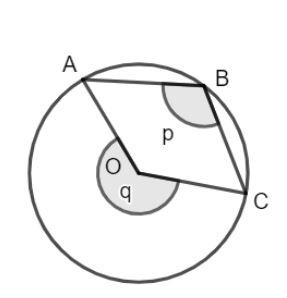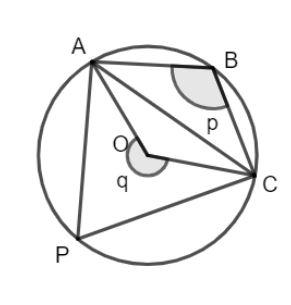QUESTION

# What is the relation between ‘p’ and ‘q’?(a). q = 2p(b). p = 2q(c). p = q(d). q > p

Hint: Draw the chord AC and draw an angle subtended by the chord AC. Then, use the properties of the cyclic quadrilateral and angle subtended by the chord to find the relation between p and q.
Join A and C in the figure to form the chord AC. Then draw an angle subtended by the chord on the circle, say, angle APC, then, we have as shown in the figure:A cyclic quadrilateral is a quadrilateral whose vertices are on the circle. The opposite angles of a cyclic quadrilateral are supplementary, that is, their addition is 180°.
The vertices of the quadrilateral ABCP lie on the circle, hence ABCP is a cyclic quadrilateral.
The angles APC and ABC are supplementary and their addition is 180°.
$\angle APC + \angle ABC = 180^\circ ..........(1)$
We know that the angle subtended by a chord on any point on the circle is twice the angle subtended at the center.
Hence, we have the value of the angle AOC as twice the value of the angle APC.
$\angle AOC = 2\angle APC$
$\angle APC = \dfrac{1}{2}\angle AOC...........(2)$
Substituting equation (2) in equation (1), we have:
$\dfrac{1}{2}\angle AOC + \angle ABC = 180^\circ$
Solving for the angle ABC, we have:
$\angle ABC = 180^\circ - \dfrac{1}{2}\angle AOC$
$2\angle ABC = 360^\circ - \angle AOC$
Angle ABC is given as angle p and angle (360° – AOC) is angle q.
$2p = q$
Hence, the correct answer is option (a).
Note: You can also directly use the property of angle subtended by a chord to find the relation between q and p but here the angles are different than usual.